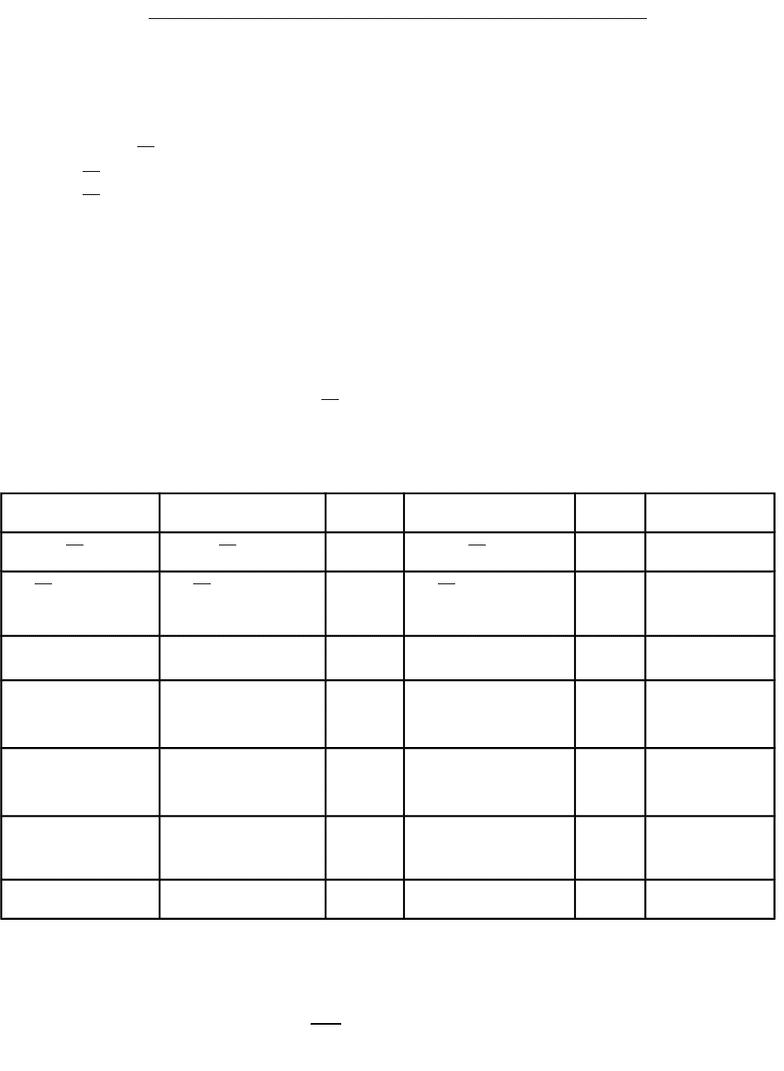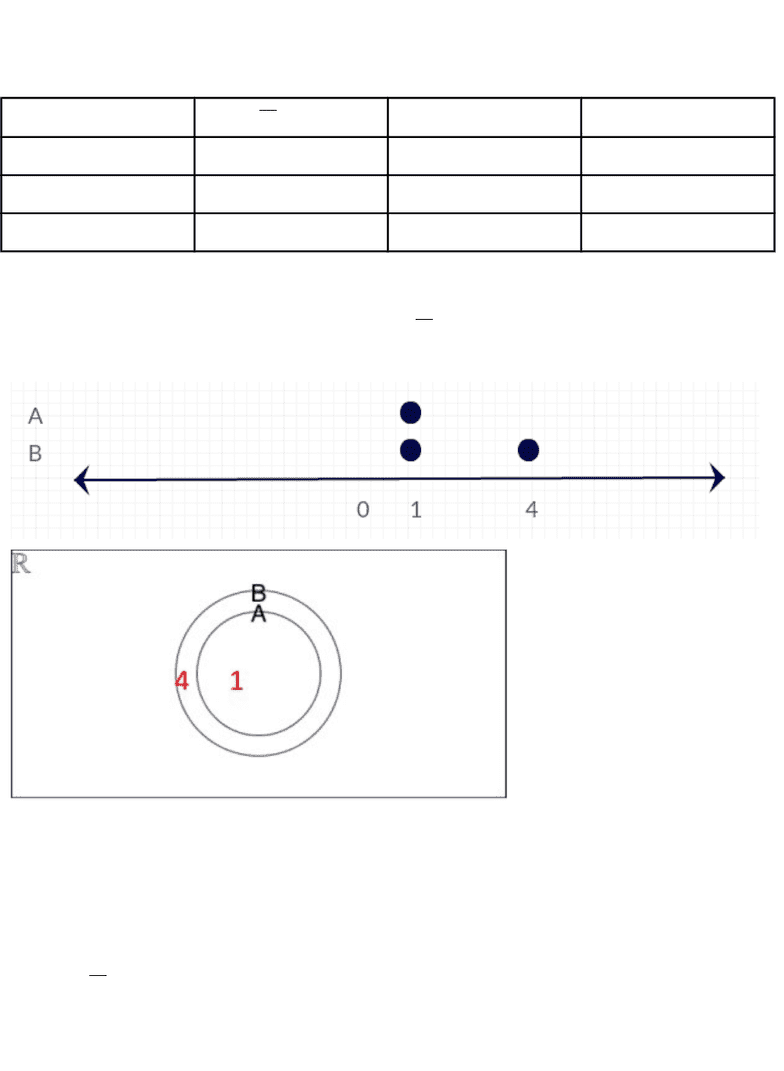Class Notes (1,000,000)
CA (610,000)
UTSG (50,000)
CSC (1,000)
CSC165H1 (200)
Lecture 3

CSC165H1 Lecture Notes - Lecture 3: Elementary Arithmetic, Solution Set, Ion

Department
Computer Science
Course Code
CSC165H1
Professor
Ilir Dema
Lecture
3

This preview shows page 1. to view the full 5 pages of the document.CSC165H1 - Lecture 3 - Universally Quantified Implication
(Continued from Lecture 2)
Our solution is the following:
Let be a real number.
Assume +
= 2.
Then
= 2 −
x
.
Then
¿
¿
¿
=
(2x)2
.
Then =
(2x)2
.
Then = 4 − + 4
x2
.
Then 0 = 4 − +5
x2
.
Then 0 = − 1) − 4). ( (
Then = 1 or = 4.
Therefore, for any number : if +
= 2 then = 1 or = 4].[
We trace our solution using a tracing table.
If = 1 If = 4 Solution Set
+
= 2 1+
= 2? True 4 +
= 2? False 1
= 2 −
x
= 2 − 1? True
= 2 −
4
?
False 1
=
(2x)2
1 =
?True 4 =
(24)2
?True 1 or 4
= 4 − + 4
x2
1 = 4 − 4(1) +
12
?
True 4 = 4 − 4(4) +
42
?
True 1 or 4
0 = 4 − +5
x2
0 = 4 − 5(1) +
12
?
True 0 = 4 − 5(4) +
42
?
True 1 or 4
0 = − 1) − 4)( ( 0 = (1 − 1)(1 −
4)?
True 0 = (4 − 1)(4 −
4)?
True 1 or 4
= 1 or = 4 1 = 1 or 1 = 4? True 4 = 1 or 4 = 4? True 1 or 4
As we trace our solution, for it to be consistent the solution set has to stay the same or get bigger.
From our tracing table we can see that the solution gets bigger, which is consistent with the
consecutive pairs of lines representing true UQI’s and the “Then” statements used to connect them.

Unlock to view full version

Only page 1 are available for preview. Some parts have been intentionally blurred.Proof Process
+
= 2? = 1 or = 4]?[
1 True True Okay
4 False True Irrelevant
0 False False Irrelevant
Using a number line and a Venn diagram…
A represents the set of all numbers such that +
= 2
B represents the set of all numbers such that = 1 or = 4.
From the number line and the Venn diagram, we can see that A is completely contained in B but B is
not contained in A, because there are more elements in B than in A.
Understanding the Solution
Every step in the solution uses a known true Universally Quantified Implication. The first ‘Then’ part :
“Then
= 2 −
x
.”, uses a known true UQI, specifically:
For all numbers and and : if = then = c.
From this known one, we can deduce: